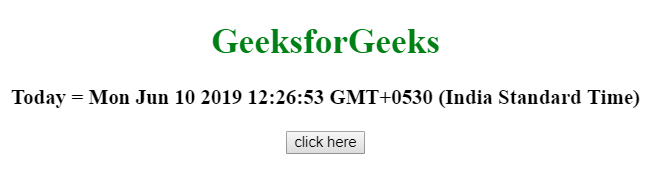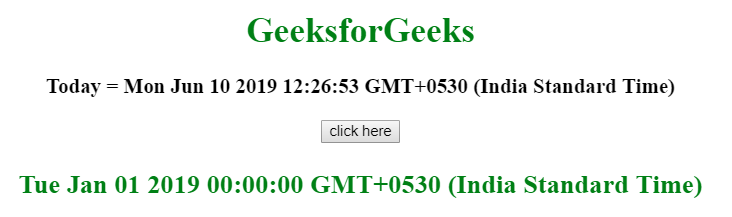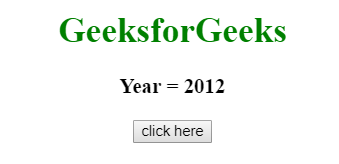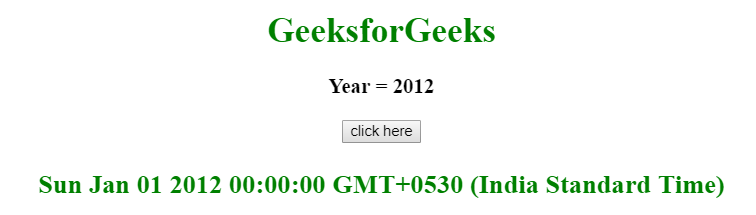Related Articles

# How to get the first day of the year in JavaScript ?

• Last Updated : 10 Jun, 2019

Given a date/year and the task is to get the first day of the year using JavaScript.

Approach 1:

• Use getFullYear() Method to get the year from given date.
• Use the new Date() function to create the new date object using year, month and day.

Example: This example uses the getFullYear() method to get the full year of the current day and then get the first day of that year.

 ` ``<``html``> ``    ``<``head``> ``        ``<``title``> ``            ``How to get the first day of``            ``the year in JavaScript ?``        ````    `` ``     ` `    ``<``body` `style` `= ``"text-align:center;"``> ``         ` `        ``<``h1` `style` `= ``"color:green;"` `> ``            ``GeeksForGeeks ``        ````         ` `        ``<``p` `id` `= ``"GFG_UP"` `style` `=``            ``"font-size: 19px; font-weight: bold;"``>``        ````         ` `        ``<``button` `onClick` `= ``"GFG_Fun()"``>``            ``click here``        ````         ` `        ``<``p` `id` `= ``"GFG_DOWN"` `style` `=``            ``"color: green; font-size: 24px; font-weight: bold;"``>``        ````         ` `        ``<``script``>``            ``var up = document.getElementById('GFG_UP');``            ``var down = document.getElementById('GFG_DOWN');``             ` `            ``var date = new Date();``     ` `            ``up.innerHTML = "Today = "+ date;``             ` `            ``function GFG_Fun() {``                 ` `                ``// Use Date(year, month, day) function``                ``down.innerHTML = new Date(date.getFullYear(), 0, 1);``            ``}``        `` ``    `` ``                    `

Output:

• Before clicking on the button:• After clicking on the button:Approach 2:

• Initialize the year to the variable (year = 2012).
• Use new Date() function to create the new date object using year, month and day.

Example: This example uses the year 2012 and then get the first day of that year.

 ` ``<``html``> ``    ``<``head``> ``        ``<``title``> ``            ``How to get the first day of``            ``the year in JavaScript ?``        ````    `` ``     ` `    ``<``body` `style` `= ``"text-align:center;"``> ``         ` `        ``<``h1` `style` `= ``"color:green;"` `> ``            ``GeeksForGeeks ``        ````         ` `        ``<``p` `id` `= ``"GFG_UP"` `style` `=``            ``"font-size: 19px; font-weight: bold;"``>``        ````         ` `        ``<``button` `onClick` `= ``"GFG_Fun()"``>``            ``click here``        ````         ` `        ``<``p` `id` `= ``"GFG_DOWN"` `style` `=``            ``"color: green; font-size: 24px; font-weight: bold;"``>``        ````         ` `        ``<``script``>``            ``var up = document.getElementById('GFG_UP');``            ``var down = document.getElementById('GFG_DOWN');``             ` `            ``// Declare year and initialize it``            ``var year = 2012;``             ` `            ``up.innerHTML = "Today's year = "+ year;``             ` `            ``function GFG_Fun() {``                 ` `                ``// Use Date(year, month, day) function``                ``// to get the first day of year``                ``down.innerHTML = new Date(year, 0, 1);``            ``}``        `` ``    `` ``                    `

Output:

• Before clicking on the button:• After clicking on the button:Hey geek! The constant emerging technologies in the world of web development always keeps the excitement for this subject through the roof. But before you tackle the big projects, we suggest you start by learning the basics. Kickstart your web development journey by learning JS concepts with our JavaScript Course. Now at it’s lowest price ever!

My Personal Notes arrow_drop_up